Search

About 178 Search Results Matching Types of Worksheet, Worksheet Section, Generator, Generator Section, Grades matching 4th Grade, Similar to Spring Word Scramble Worksheet, Page 4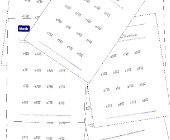Simple Division Worksheets

This group of worksheets makes a good introduction...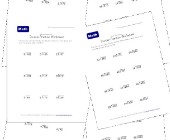Division Practice Worksheets

This collection of division practice worksheets is...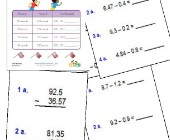Subtracting Decimals Worksheets

These worksheets will help children practice decim...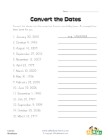Convert the Dates Worksheet

Convert the dates into the numerical format: month...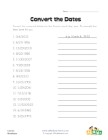Convert the Dates Worksheet

Convert the numerical dates into the format: month...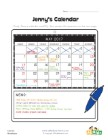Reading a Calendar Worksheet

Answer the questions using the calendar as a refer...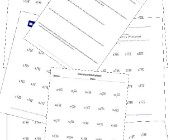Division Worksheets

Help kids learn division with this collection of m...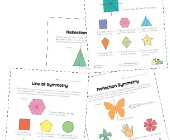Symmetry Worksheets

Help kids learn about the concept of symmetry with...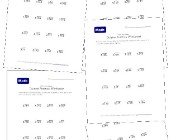Simple Division Worksheets with Remainders

This section of division worksheets is dedicated t...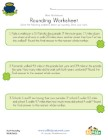St. Patrick's Day Rounding Worksheet

Practice rounding with a fun St. Patrick's Day the...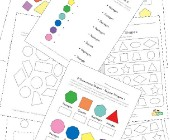Geometry Worksheets

Help kids learn the early basics of geometry inclu...